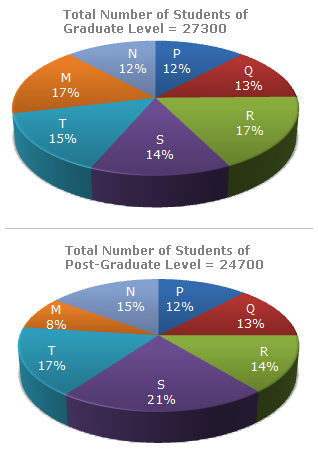# Data Interpretation - Pie Charts - Discussion

The following pie-charts show the distribution of students of graduate and post-graduate levels in seven different institutes in a town.3.

How many students of institutes of M and S are studying at graduate level?

 [A]. 7516 [B]. 8463 [C]. 9127 [D]. 9404

Explanation:

Students of institute M at graduate level= 17% of 27300 = 4641.

Students of institute S at graduate level = 14% of 27300 = 3822.Total number of students at graduate in institutes M and S = (4641 + 3822) = 8463.